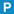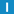# class LocalDateTime

Library: Foundation
Package: DateTime

## Description

This class represents an instant in local time (as opposed to UTC), expressed in years, months, days, hours, minutes, seconds and milliseconds based on the Gregorian calendar.

In addition to the date and time, the class also maintains a time zone differential, which denotes the difference in seconds from UTC to local time, i.e. UTC = local time - time zone differential.

Although LocalDateTime supports relational and arithmetic operators, all date/time comparisons and date/time arithmetic should be done in UTC, using the DateTime or Timestamp class for better performance. The relational operators normalize the dates/times involved to UTC before carrying out the comparison.

The time zone differential is based on the input date and time and current time zone. A number of constructors accept an explicit time zone differential parameter. These should not be used since daylight savings time processing is impossible since the time zone is unknown. Each of the constructors accepting a tzd parameter have been marked as deprecated and may be removed in a future revision.

## Constructors

### LocalDateTime

Creates a LocalDateTime with the current date/time for the current time zone.

### LocalDateTime

LocalDateTime(
const DateTime & dateTime
);

Creates a LocalDateTime from the UTC time given in dateTime, using the time zone differential of the current time zone.

### LocalDateTime

LocalDateTime(
double julianDay
);

Creates a LocalDateTime for the given Julian day in the local time zone.

### LocalDateTime

LocalDateTime(
const LocalDateTime & dateTime
);

Copy constructor. Creates the LocalDateTime from another one.

### LocalDateTime

LocalDateTime(
int tzd,
const DateTime & dateTime
);

Deprecated. This function is deprecated and should no longer be used.

Creates a LocalDateTime from the UTC time given in dateTime, using the given time zone differential. Adjusts dateTime for the given time zone differential.

### LocalDateTime

LocalDateTime(
int tzd,
double julianDay
);

Deprecated. This function is deprecated and should no longer be used.

Creates a LocalDateTime for the given Julian day in the time zone denoted by the time zone differential in tzd.

### LocalDateTime

LocalDateTime(
int tzd,
const DateTime & dateTime,
);

Deprecated. This function is deprecated and should no longer be used.

Creates a LocalDateTime from the UTC time given in dateTime, using the given time zone differential. If adjust is true, adjusts dateTime for the given time zone differential.

### LocalDateTime

LocalDateTime(
int year,
int month,
int day,
int hour = 0,
int minute = 0,
int second = 0,
int millisecond = 0,
int microsecond = 0
);

Creates a LocalDateTime for the given Gregorian local date and time.

• year is from 0 to 9999.
• month is from 1 to 12.
• day is from 1 to 31.
• hour is from 0 to 23.
• minute is from 0 to 59.
• second is from 0 to 60 (allowing leap seconds).
• millisecond is from 0 to 999.
• microsecond is from 0 to 999.

### LocalDateTime

LocalDateTime(
int tzd,
int year,
int month,
int day,
int hour,
int minute,
int second,
int millisecond,
int microsecond
);

Deprecated. This function is deprecated and should no longer be used.

Creates a LocalDateTime for the given Gregorian date and time in the time zone denoted by the time zone differential in tzd.

• tzd is in seconds.
• year is from 0 to 9999.
• month is from 1 to 12.
• day is from 1 to 31.
• hour is from 0 to 23.
• minute is from 0 to 59.
• second is from 0 to 60 (allowing leap seconds).
• millisecond is from 0 to 999.
• microsecond is from 0 to 999.

### LocalDateTimeLocalDateTime(
Timestamp::UtcTimeVal utcTime,
Timestamp::TimeDiff diff,
int tzd
);

## Destructor

### ~LocalDateTime

~LocalDateTime();

Destroys the LocalDateTime.

## Member Functions

### assign

LocalDateTime & assign(
int year,
int month,
int day,
int hour = 0,
int minute = 0,
int second = 0,
int millisecond = 0,
int microseconds = 0
);

Assigns a Gregorian local date and time.

• year is from 0 to 9999.
• month is from 1 to 12.
• day is from 1 to 31.
• hour is from 0 to 23.
• minute is from 0 to 59.
• second is from 0 to 60 (allowing leap seconds).
• millisecond is from 0 to 999.
• microsecond is from 0 to 999.

### assign

LocalDateTime & assign(
int tzd,
int year,
int month,
int day,
int hour,
int minute,
int second,
int millisecond,
int microseconds
);

Deprecated. This function is deprecated and should no longer be used.

Assigns a Gregorian local date and time in the time zone denoted by the time zone differential in tzd.

• tzd is in seconds.
• year is from 0 to 9999.
• month is from 1 to 12.
• day is from 1 to 31.
• hour is from 0 to 23.
• minute is from 0 to 59.
• second is from 0 to 60 (allowing leap seconds).
• millisecond is from 0 to 999.
• microsecond is from 0 to 999.

### assign

LocalDateTime & assign(
int tzd,
double julianDay
);

Deprecated. This function is deprecated and should no longer be used.

Assigns a Julian day in the time zone denoted by the time zone differential in tzd.

### dayint day() const;

Returns the day within the month (1 to 31).

### dayOfWeekint dayOfWeek() const;

Returns the weekday (0 to 6, where 0 = Sunday, 1 = Monday, ..., 6 = Saturday).

### dayOfYearint dayOfYear() const;

Returns the number of the day in the year. January 1 is 1, February 1 is 32, etc.

### hourint hour() const;

Returns the hour (0 to 23).

### hourAMPMint hourAMPM() const;

Returns the hour (0 to 12).

### isAMbool isAM() const;

Returns true if hour < 12;

### isPMbool isPM() const;

Returns true if hour >= 12.

### julianDaydouble julianDay() const;

Returns the Julian day for the date.

### microsecondint microsecond() const;

Returns the microsecond (0 to 999)

### millisecondint millisecond() const;

Returns the millisecond (0 to 999)

### minuteint minute() const;

Returns the minute (0 to 59).

### monthint month() const;

Returns the month (1 to 12).

### operator !=

bool operator != (
const LocalDateTime & dateTime
) const;

### operator +

LocalDateTime operator + (
const Timespan & span
) const;

### operator +=

LocalDateTime & operator += (
const Timespan & span
);

### operator -

LocalDateTime operator - (
const Timespan & span
) const;

### operator -

Timespan operator - (
const LocalDateTime & dateTime
) const;

### operator -=

LocalDateTime & operator -= (
const Timespan & span
);

### operator <

bool operator < (
const LocalDateTime & dateTime
) const;

### operator <=

bool operator <= (
const LocalDateTime & dateTime
) const;

### operator =

LocalDateTime & operator = (
const LocalDateTime & dateTime
);

Assigns another LocalDateTime.

### operator =

LocalDateTime & operator = (
const Timestamp & timestamp
);

Assigns a timestamp

### operator =

LocalDateTime & operator = (
double julianDay
);

Assigns a Julian day in the local time zone.

### operator ==

bool operator == (
const LocalDateTime & dateTime
) const;

### operator >

bool operator > (
const LocalDateTime & dateTime
) const;

### operator >=

bool operator >= (
const LocalDateTime & dateTime
) const;

### secondint second() const;

Returns the second (0 to 59).

### swap

void swap(
LocalDateTime & dateTime
);

Swaps the LocalDateTime with another one.

### timestampTimestamp timestamp() const;

Returns the date and time expressed as a Timestamp.

### tzdint tzd() const;

Returns the time zone differential.

### utc

DateTime utc() const;

Returns the UTC equivalent for the local date and time.

### utcTimeTimestamp::UtcTimeVal utcTime() const;

Returns the UTC equivalent for the local date and time.

### weekint week(
int firstDayOfWeek = DateTime::MONDAY
) const;

Returns the week number within the year. FirstDayOfWeek should be either SUNDAY (0) or MONDAY (1). The returned week number will be from 0 to 53. Week number 1 is the week containing January 4. This is in accordance to ISO 8601.

The following example assumes that firstDayOfWeek is MONDAY. For 2005, which started on a Saturday, week 1 will be the week starting on Monday, January 3. January 1 and 2 will fall within week 0 (or the last week of the previous year).

For 2007, which starts on a Monday, week 1 will be the week starting on Monday, January 1. There will be no week 0 in 2007.

### yearint year() const;

Returns the year.

### adjustForTzdAdjust the _dateTime member based on the _tzd member.

### determineTzdvoid determineTzd(